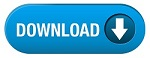Home » SSC » Model Questions for Scientific Assistant (IMD) Exam (Paper-2) PHYSICS – Set 28

# Model Questions for Scientific Assistant (IMD) Exam (Paper-2) PHYSICS – Set 28

## 1. An electric circuit where the resistors are connected in such a manner that the current (in amperes) flowing through each resistor has to be the same as the current flowing through each of the other resistors.

a. Alternating current (AC)
b. Electric power
c. Series ciruit
d. Parallel circuit

## 2. An electric circuit where the resistors are connected in such a manner that the voltage (potential) across each resistor has to be the same as the voltage across each of the other resistors.

a. Alternating current (AC)
b. Electric power
c. Series ciruit
d. Parallel circuit

## 3. Measured in Watts or kilowatts. The amount of electrical energy transferred per unit time.

a. Alternating current (AC)
b. Electric power
c. Series ciruit
d. Parallel circuit

## 4. A magnetic field runs from the top of this page down to the bottom of this page. An electron is fired from left to right across the page. The electron is ___ by the magnetic field.

a. Indifferent to
b. attracted by
c. repelled by
d. deflected out of the plane of the page

## 5. A small piece of metal is attracted to a large magnet. The magnet is attracted to the small piece of metal with a force that is ____ compared to the force with which the metal is attracted to the magnet.

a. very large
b. very small
c. the same
d. similar but not the same

#a. zero
b. infinite
c. large
d. small

## 7. Magnetism is caused by—–

a. pieces of gold
b. pieces of copper
c. moving magnetic charges
d. moving electric charges

## 8. Some of the planets in our Solar system have magnetic fields. The likely cause of these magnetic fields is—-

a. moving electric charges in the core of the planets
b. pieces of iron on the planets
c. pieces of gold on the planets
d. pieces of copper on the planets

## 9. This machine converts mechanical energy into electrical energy. A coil rotates inside a magnetic field, and current is induced in the coil.

a. Electromagnetic Induction
c. Generator
d. Transformer

## 10. A magnetic field changes with time, and generates an electric field. If the magnetic field changes faster, the induced electric field is stronger.

a. Electromagnetic Induction
c. Generator
d. Transformer

#